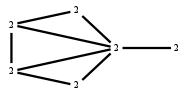# The Markov Bases Database

## G136g_bin

The binary graphical model of G136.

It is a hierarchical model of 6 variables. The dimension of the model is 17.The cardinality of the statespace is 64.

### Properties of the Markov basis

 Markov degree 2 360

 degree 2 360

The model has the following properties:

• All variables are binary.
• It is a graphical model.
• The semigroup is normal.### FILES

 Markov basis: sufficient statistics matrix: G136g_bin.mar (67.86 kb) G136g_bin.mat (3.53 kb) G136g_bin.mod (47 b) G136g_bin.tar.gz (8.08 kb)